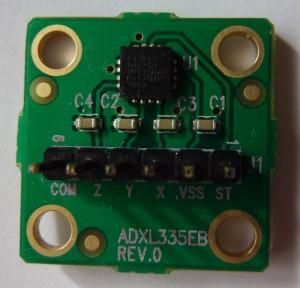# Simple angle meter using ADXL335 accelerometer [Arduino]ADXL335 is 3 axis accelerometer with analog output from Analog Devices. You can buy it as an evaluation kit  with standard 2,5 mm connector.

ADXL335 acceleration measurement range is +/- 3 g. Supply voltage is 1.8 –  3.6 V, however all specifications at the datasheet is given at 3.0 V. This accelerometer has  3 outputs for X,Y,Z axis which voltage is proportional to acceleration on specific axis.

At midpoint when acceleration is 0 g output is typically 1/2 of supply voltage. If a supply voltage is 3V, then output is 1.5 V. Output sensitivity typically is 300 mV/g.